Next: Manipulating Points and Up: Important concepts from projective Previous: Important concepts from projective

# Homogeneous Coordinates

The equations for perspective projection to the image plane are non-linear when expressed in non-homogeneous coordinates, but are linear in homogeneous coordinates. This is characteristic of all transformations in projective geometry, not just perspective projection. It provides one of the main motivations for the use of homogeneous coordinates, since linear systems are symbolically and numerically easier to handle than non-linear ones.

The non-homogeneous coordinates of an image point are a 2-vector, while the homogeneous coordinates are a 3-vector. The simplest way to assign the third component is to make it equal to one,An important property of homogeneous coordinates is that a pair of homogeneous vectors are equivalent if one is a scalar multiple of the other,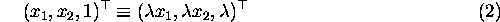where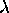is a scalar,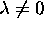.

This equivalence is plain when one considers the transformation from homogeneous coordinates back to image plane coordinates, which is carried out by reversing the process for converting into homogeneous coordinates - scale the homogeneous vector such that the third component is equal to one and then discard the third component. For the homogeneous vector in Equation 1,and for the equivalent homogeneous vector in Equation 2,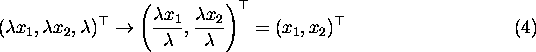so all equivalent homogeneous coordinates correspond to the same non-homogeneous coordinates as expected.

Image lines are also represented by 3-vectors in homogeneous coordinates. The homogeneous pointswhich lie on the homogeneous line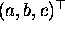are specified by the equation,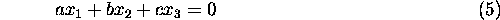As was the case for points, homogeneous coordinates for lines are equivalent if one is a scalar multiple of the other.

In addition to the linearity which they bring to equations, a further important benefit of using homogeneous coordinates is that they make it possible to represent points which are at infinity on the image plane. In non-homogeneous coordinates, there is no numerical representation for a point at infinity; in homogeneous coordinates, such a point has its third component equal to zero i.e. it has the form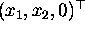. Points at infinity are called ideal points. The commonest way in which an ideal point arises in practice is as the intersection point of two parallel lines. Note that having obtained a representation for ideal points, there is no need to treat them differently from any other point on the image plane - the manipulations and transformations of projective geometry apply equally well to all points both non-ideal and ideal. When the set of non-ideal and ideal points is taken together, the result is called a projective plane.Next: Manipulating Points and Up: Important concepts from projective Previous: Important concepts from projective

Bob Fisher
Fri Nov 7 12:08:26 GMT 1997